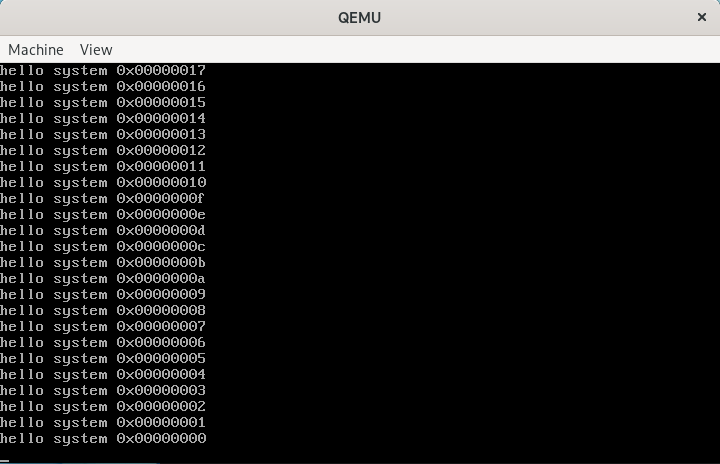• 累计撰写 36 篇文章
• 累计收到 19 条评论

# 操作系统实现-printk2022-05-11 / 0 评论 / 46 阅读 / 正在检测是否收录...

`%[flags][width][.prec][h|l|L][type]`

• `%`：格式引入字符
• `flags`：可选的标志字符序列
• `width`：可选的宽度指示符
• `.prec`：可选的精度指示符
• `h|l|L`：可选的长度修饰符
• `type`：转换类型

• `va_list`：保存可变参数指针
• `va_start`：启用可变参数
• `va_arg`：获取下一个参数
• `va_end`：结束可变参数

``````typedef char *va_list; // 保存可变参数
// 因为是将参数全部压栈，最后一个压栈的是参数的个数，所以只需要挨着在内存中找多少个就知道了
#define va_start(ap, v) (ap = (va_list)&v + sizeof(char *)) // 启用可变参数 指向v下一个参数地址
#define va_arg(ap, t) (*(t *)((ap += sizeof(char *)) - sizeof(char *))) // 获取下一个参数 并将值转换为t格式
#define va_end(ap) (ap = (va_list)0) // 结束可变参数``````

``````void kernel_init()
{
console_init();
int cnt = 30;
while (cnt--)
{
printk("hello system %#010x\n", cnt);
}
}````````````static char buf;

int printk(const char *fmt, ...)
{
va_list args;
int i;
// 此时的args就是fmt下一个参数的地址
va_start(args, fmt);
// 将内容格式化到buf里面
i = vsprintf(buf, fmt, args);

va_end(args);
// 写到控制台上
console_write(buf, i);

return i;
}``````

``printk("hello system %#010x\n", 29);``

``````int vsprintf(char *buf, const char *fmt, va_list args)
{
int len;
int i;

// 用于存放转换过程中的字符串
char *str;
char *s;
int *ip;

// number() 函数使用的标志
int flags;

int field_width; // 输出字段宽度
int precision;   // min 整数数字个数；max 字符串中字符个数
int qualifier;   // 'h', 'l' 或 'L' 用于整数字段

// 首先将字符指针指向 buf
// 然后扫描格式字符串，
// 对各个格式转换指示进行相应的处理
for (str = buf; *fmt; ++fmt)
{
// 格式转换指示字符串均以 '%' 开始
// 这里从 fmt 格式字符串中扫描 '%'，寻找格式转换字符串的开始
// 不是格式指示的一般字符均被依次存入 str
if (*fmt != '%')
{
*str++ = *fmt;
continue;
}

// 下面取得格式指示字符串中的标志域，并将标志常量放入 flags 变量中
flags = 0;
repeat:
// 掉过第一个 %
++fmt;
switch (*fmt)
{
// 左对齐调整
case '-':
flags |= LEFT;
goto repeat;
// 放加号
case '+':
flags |= PLUS;
goto repeat;
// 放空格
case ' ':
flags |= SPACE;
goto repeat;
// 是特殊转换
case '#':
flags |= SPECIAL;
goto repeat;
// 要填零(即'0')，否则是空格
case '0':
goto repeat;
}

// 取当前参数字段宽度域值，放入 field_width 变量中
field_width = -1;

// 如果宽度域中是数值则直接取其为宽度值
if (is_digit(*fmt))
field_width = skip_atoi(&fmt);

// 如果宽度域中是字符 '*'，表示下一个参数指定宽度
else if (*fmt == '*')
{
++fmt;
// 因此调用 va_arg 取宽度值
field_width = va_arg(args, int);

// 若此时宽度值小于 0，则该负数表示其带有标志域 '-' 标志（左对齐）
if (field_width < 0)
{
// 因此还需在标志变量中添入该标志，并将字段宽度值取为其绝对值
field_width = -field_width;
flags |= LEFT;
}
}

// 取格式转换串的精度域，并放入 precision 变量中
precision = -1;

// 精度域开始的标志是'.' 其处理过程与上面宽度域的类似
if (*fmt == '.')
{
++fmt;
// 如果精度域中是数值则直接取其为精度值
if (is_digit(*fmt))
precision = skip_atoi(&fmt);

// 如果精度域中是字符'*'，表示下一个参数指定精度
else if (*fmt == '*')
{
// 因此调用 va_arg 取精度值
precision = va_arg(args, int);
}
// 若此时宽度值小于 0，则将字段精度值取为其绝对值
if (precision < 0)
precision = 0;
}

// 下面这段代码分析长度修饰符，并将其存入 qualifer 变量
qualifier = -1;
if (*fmt == 'h' || *fmt == 'l' || *fmt == 'L')
{
qualifier = *fmt;
++fmt;
}

// 下面分析转换指示符
switch (*fmt)
{

// 如果转换指示符是'c'，则表示对应参数应是字符
case 'c':
// 此时如果标志域表明不是左对齐，
if (!(flags & LEFT))
// 则该字段前面放入 (宽度域值 - 1) 个空格字符，然后再放入参数字符
while (--field_width > 0)
*str++ = ' ';
*str++ = (unsigned char)va_arg(args, int);
// 如果宽度域还大于 0，则表示为左对齐
// 则在参数字符后面添加 (宽度值-1) 个空格字符
while (--field_width > 0)
*str++ = ' ';
break;

// 如果转换指示符是 's'，则表示对应参数是字符串
case 's':
s = va_arg(args, char *);
// 首先取参数字符串的长度
len = strlen(s);
// 若其超过了精度域值, 则扩展精度域=字符串长度
if (precision < 0)
precision = len;
else if (len > precision)
len = precision;

// 此时如果标志域表明不是左对齐
if (!(flags & LEFT))
// 则该字段前放入 (宽度值-字符串长度) 个空格字符
while (len < field_width--)
*str++ = ' ';
// 然后再放入参数字符串
for (i = 0; i < len; ++i)
*str++ = *s++;
// 如果宽度域还大于 0，则表示为左对齐
// 则在参数字符串后面，添加(宽度值-字符串长度)个空格字符
while (len < field_width--)
*str++ = ' ';
break;

// 如果格式转换符是'o'，表示需将对应的参数转换成八进制数的字符串
case 'o':
str = number(str, va_arg(args, unsigned long), 8,
field_width, precision, flags);
break;

// 如果格式转换符是'p'，表示对应参数的一个指针类型
case 'p':
// 此时若该参数没有设置宽度域，则默认宽度为 8，并且需要添零
if (field_width == -1)
{
field_width = 8;
}
str = number(str,
(unsigned long)va_arg(args, void *), 16,
field_width, precision, flags);
break;

// 若格式转换指示是 'x' 或 'X'
// 则表示对应参数需要打印成十六进制数输出
case 'x':
// 'x'表示用小写字母表示
flags |= SMALL;
case 'X':
str = number(str, va_arg(args, unsigned long), 16,
field_width, precision, flags);
break;

// 如果格式转换字符是'd', 'i' 或 'u'，则表示对应参数是整数
case 'd':
case 'i':
// 'd', 'i'代表符号整数，因此需要加上带符号标志
flags |= SIGN;
// 'u'代表无符号整数
case 'u':
str = number(str, va_arg(args, unsigned long), 10,
field_width, precision, flags);
break;

// 若格式转换指示符是 'n'
// 表示要把到目前为止转换输出的字符数保存到对应参数指针指定的位置中
case 'n':
// 首先利用 va_arg() 取得该参数指针
ip = va_arg(args, int *);
// 然后将已经转换好的字符数存入该指针所指的位置
*ip = (str - buf);
break;

default:
// 若格式转换符不是 '%'，则表示格式字符串有错
if (*fmt != '%')
// 直接将一个 '%' 写入输出串中
*str++ = '%';
// 如果格式转换符的位置处还有字符，则也直接将该字符写入输出串中
// 然后继续循环处理格式字符串
if (*fmt)
*str++ = *fmt;
else
// 否则表示已经处理到格式字符串的结尾处，则退出循环
--fmt;
break;
}
}
// 最后在转换好的字符串结尾处添上字符串结束标志
*str = '\0';

// 返回转换好的字符串长度值
i = str - buf;
assert(i < 1024);
return i;
}``````

0